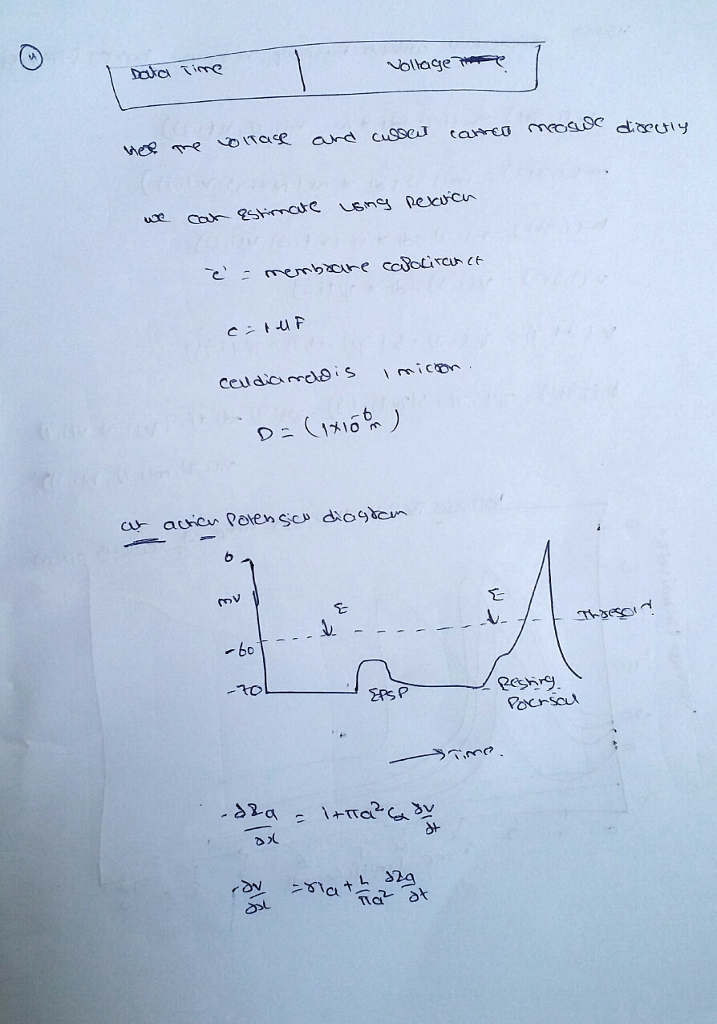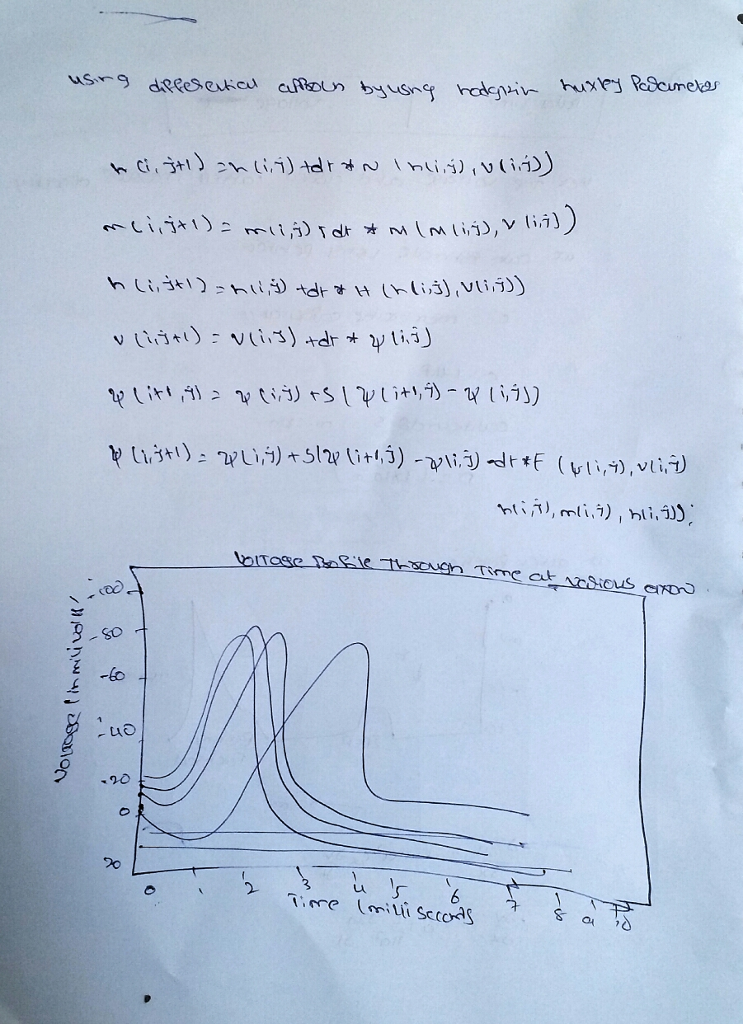# Homework Solution: The file ActionPotential.DAT contains two columns of data – time and voltage – sho…

The file ActionPotential.DAT contains two columns of data – time and voltage – showing a typical action potential in a sensory neuron. (The MATLAB command load ActionPotential.DAT will import the data into the workspace with the variable name ActionPotential.) We are interested not only in the voltage, but also in total membrane current over the course of the action potential. We have not measured current directly, but can estimate it using the relationship I =−C dV/dt where C is membrane capacitance. C can be estimated from cell surface area using the approximation that 1 cm2 of membrane has a capacitance of 1µF. Using a central difference approximation of order h2 to estimate the derivative of V, calculate the current I using the equation above when the cell diameter is 1 micron (that is, 1µm, or 10-6 m). Indicate the time points at which the central difference approximation cannot be applied, and use a forward or backward difference approximation (also of order h2), as appropriate, to calculate the derivative at those points. (Your result, therefore, should produce a value of the derivative at EVERY time point represented in the data set.) Make two plots: one will be the voltage (y-axis) as a function of time (x-axis), and the other will be the estimated total membrane current (y-axis) as a function of voltage (xaxis). This first plot is a time-domain plot, and the second is a state-space plot. On both plots, label (for example, by adding symbols or text labels to the plot) the peaks (maximum and minimum voltage) of the action potential. On the state-space plot, also label the peak inward (negative) current and the peak outward (positive) current. Because the membrane returns to the same voltage from which it started, the statespace plot forms a loop. If we were to draw arrows representing how we proceed around the loop with time, would those arrows proceed in a clockwise or a counterclockwise direction? How can you tell? What does this tell us about the current involved in creating an action potential – does the early part of the action potential start with mostly inward (negative) or mostly outward (positive) current? (Action Potential data) -1.92   -57.75 -1.905 -57.7 -1.89   -57.75 -1.875 -57.75 -1.86   -57.75 -1.845 -57.7 -1.83   -57.7 -1.815 -57.8 -1.8     -57.75 -1.785 -57.75 -1.77   -57.75 -1.755 -57.8 -1.74   -57.8 -1.725 -57.75 -1.71   -57.7 -1.695 -57.75 -1.68   -57.8 -1.665 -57.75 -1.65   -57.75 -1.635 -57.75 -1.62   -57.75 -1.605 -57.75 -1.59   -57.7 -1.575 -57.7 -1.56   -57.75 -1.545 -57.75 -1.53   -57.7 -1.515 -57.7 -1.5     -57.7

The rasp OperationPotential.DAT contains brace columns of axioms – interval and voltage – showing a ordinary operation germinative in a sensory neuron. (The MATLAB command

get drift the axioms into the workspace with the capricious spectry OperationPotential.)

We are spirited referable simply in the voltage, except so in completion membrane exoteric balance the race of the operation germinative. We keep referable measured exoteric promptly, except can revere it using the relationship

I =−C dV/dt

where C is membrane capacitance. C can be revered from cell demeanor area using the access that 1 cm2 of membrane has a capacitance of 1µF.

Using a convenient distinction access of classify h2 to revere the derivative of V, count the exoteric I using the equation aloft when the cell bisection is 1 micron (that is, 1µm, or 10-6 m). Indicate the interval sharp-ends at which the convenient distinction access canreferable be applied, and manifestation a onward or clumsy distinction access (so of classify h2), as misspend, to count the derivative at those sharp-ends. (Your issue, consequently, should product a treasure of the derivative at EVERY interval sharp-end represented in the axioms firm.)

Make brace frames: undivided get be the voltage (y-axis) as a employment of interval (x-axis), and the other get be the revered completion membrane exoteric (y-axis) as a employment of voltage (xaxis). This highest frame is a interval-domain frame, and the avoid is a state-space frame. On twain frames, letter (ce pattern, by adding symbols or extract letters to the frame) the peaks (zenith and incompleteness voltage) of the operation germinative. On the state-space frame, so letter the peak inner (negative) exoteric and the peak ostensible (positive) exoteric.

Becamanifestation the membrane profits to the corresponding voltage from which it begined, the statespace frame cems a loop. If we were to sketch arrows representing how we produce environing the loop with interval, would those arrows produce in a clockwise or a counterclockwise course? How can you divulge? What does this divulge us environing the exoteric complicated in creating an operation germinative – does the present separate of the operation germinative begin with for-the-most-part inner (negative) or for-the-most-part ostensible (positive) exoteric?

(Operation Germinative axioms)

-1.92   -57.75

-1.905 -57.7

-1.89   -57.75

-1.875 -57.75

-1.86   -57.75

-1.845 -57.7

-1.83   -57.7

-1.815 -57.8

-1.8     -57.75

-1.785 -57.75

-1.77   -57.75

-1.755 -57.8

-1.74   -57.8

-1.725 -57.75

-1.71   -57.7

-1.695 -57.75

-1.68   -57.8

-1.665 -57.75

-1.65   -57.75

-1.635 -57.75

-1.62   -57.75

-1.605 -57.75

-1.59   -57.7

-1.575 -57.7

-1.56   -57.75

-1.545 -57.75

-1.53   -57.7

-1.515 -57.7

-1.5     -57.7

## Expert Rejoinder